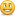Learning never exhausts the mind
Home >  Coding > C# ASP.Net MVC > Format A Number as KB, MB or GB with C#

# Format A Number as KB, MB or GB with C#

First Published 1st May 2009, Last Updated by

This short snippet can be used to pretty format a number of a file size (in bytes) into a pretty string that includes file size metric.

This snippet is a modified version of the first posting which has been refactored by Ben Laan (see comments below). The original script was copied from some other website quite a while ago, cannot remember where from, but I have used it extensively and thought I would share this useful method. If you know who the original author is please let me know.

```public string FormatBytes(long bytes)
{
const int scale = 1024;
string[] orders = new string[] { "GB", "MB", "KB", "Bytes" };
long max = (long)Math.Pow(scale, orders.Length - 1);

foreach (string order in orders)
{
if ( bytes > max )
return string.Format("{0:##.##} {1}", decimal.Divide( bytes, max ), order);

max /= scale;
}
return "0 Bytes";
}```

The original code:

```public string FormatBytes(int Bytes)
{
string filesize;
if (Bytes >= 1073741824)
{
decimal size = decimal.Divide(Bytes, 1073741824);
filesize = string.Format("{0:##.##} GB", size);
}
else if (Bytes >= 1048576)
{
decimal size = decimal.Divide(Bytes, 1048576);
filesize = string.Format("{0:##.##} MB", size);
}
else if (Bytes >= 1024)
{
decimal size = decimal.Divide(Bytes, 1024);
filesize = string.Format("{0:##.##} KB", size);
}
else if (Bytes > 0 &amp; Bytes < 1024)
{
decimal size = Bytes;
filesize = string.Format("{0:##.##} Bytes", size);
}
else
{
filesize = "0 Bytes";
}
return filesize;
}```

I also have a similar function written in PHP to pretty format file sizes.

11 thoughts on “Format A Number as KB, MB or GB with C#
•7th December 2014 at 12:00 am

I think the newer solution is slower and incorrect.

[Fact]
public void ShouldFormatGigabytes()
{
const long sut = 1 * 1024 * 1024 * 1024;
Assert.Equal("1 GB", FormatBytes(sut));
}

Actual: 1024 MB

• 31st March 2011 at 12:00 am

Another refactoring without the loop (in VB):
Private Function FormatBytes(ByVal bytes As Long) As String
Const scale = 1024
Dim orders As String() = New String() {"", "K", "M", "G", "T", "P", "E"}

Dim rank = Math.Floor(Math.Log(bytes, scale))
If rank >= orders.Length Then rank -= 1
Return String.Format("{0:##.##} {1}B", bytes / Math.Pow(scale, rank), orders(rank))
End Function

• 6th December 2010 at 12:00 am

if (bytes == max) return string.Format("1 {0}", order);

•9th March 2010 at 12:00 am

I got the ultimate solution:

return (String.Format("{0:##.##0}",
Bytes >= 1073741824 ? decimal.Divide(Bytes, 1073741824) + "GB" :
Bytes >= 1048576 ? decimal.Divide(Bytes, 1048576) + "MB" :
Bytes >= 1024 ? decimal.Divide(Bytes, 1024) + "KB" :
Bytes > 0 & Bytes < 1024 ? Bytes + "Bytes" : 0 + "0 Bytes"
));

Happy formating•22nd February 2010 at 12:00 am

So I used this in a console app recently as well, here was my function.. just to wrap it up..

static string FormatBytes(long bytes)
{
const long scale = 1024;
string[] orders = new string[]{ "YB", "ZB", "EB", "PB", "TB", "GB", "MB", "KB", "Bytes" };
var max = (long)Math.Pow(scale, (orders.Length - 1));
foreach (string order in orders)
{
if (bytes > max)
return string.Format("{0:##.##} {1}", Decimal.Divide(bytes, max), order);
max /= scale;
}
return "0 Bytes";
}

•22nd February 2010 at 12:00 am

Okay so this actually only works up to the EB's, it kind of looks like somewhere between EB's and ZB's we hit the max value for the long data type, and the previous function would return just "YB" for all of the string values. So I modified the orders array to this:

string[] orders = new string[]{ "EB", "PB", "TB", "GB", "MB", "KB", "Bytes" };

You could try a different data type, but I'm not measuring anything larger than EB's anyways.

• 11th February 2010 at 12:00 am

Hi, nice code, thanks!

I also changed this line to do a little future proofing for Diomede Storage.string[] orders = new string[] { "YB", "ZB", "EB", "PB", "TB", "GB", "MB", "KB", "Bytes" };

-- Ross

• 20th December 2012 at 12:00 am

@Ross what about Brontobytes?• 10th February 2010 at 12:00 am

thanks code worked great. if your bytes are displayed in MB like mine were all you have to do is modify the scale..mine looked something like scale = (1024/10) or you could go the other way scale = 10240

awesome code thanks a bunch.

•5th May 2009 at 12:00 am

Hi ben,

Thanks for the refactor!

I wasn't completely happy with the code, especially the hard coded byte values; however I copied if from the somewhere on the web and just carried on using it. "If it ain't broke, don't fix it".

I will probably give your version a try though!

Thanks

•5th May 2009 at 12:00 am

This code has 4 repeating blocks! Sounds like it needs a good refactoring:

public string FormatBytesNew( long bytes )
{
const int scale = 1024;

var orders = new[] { "GB", "MB", "KB", "Bytes" };
var max = (long) Math.Pow( scale, orders.Length - 1 );

foreach ( var order in orders )
{
if ( bytes > max )
return string.Format( "{0:##.##} {1}", Decimal.Divide( bytes, max ), order );

max /= scale;
}
return "0 Bytes";
}